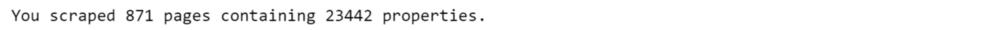1个月前 (07-01) |   抢沙发  9```headers = ({'User-Agent':
'Mozilla/5.0 (Windows NT 6.1) AppleWebKit/537.36 (KHTML, like Gecko) Chrome/41.0.2228.0 Safari/537.36'})```

```sapo = "https://casa.sapo.pt/Venda/Apartamentos/?sa=11&amp;or=10"`html_soup = BeautifulSoup(response.text, 'html.parser')``house_containers = html_soup.find_all('div', class_="searchResultProperty")`

```first = house_containers
first.find_all('span')````# setting up the lists that will form our dataframe with all the results`
```titles = []
created = []
prices = []
areas = []
zone = []
condition = []
descriptions = []
urls = []
thumbnails = []

```

```%%time
n_pages = 0
for page in range(0,900):
n_pages += 1
sapo_url = 'https://casa.sapo.pt/Venda/Apartamentos/?sa=11&amp;lp=10000&amp;or=10'+'&amp;pn='+str(page)
page_html = BeautifulSoup(r.text, 'html.parser')
house_containers = page_html.find_all('div', class_="searchResultProperty")
if house_containers != []:
for container in house_containers:

# Price
price = container.find_all('span').text
if price == 'Contacte Anunciante':
price = container.find_all('span').text
if price.find('/') != -1:
price = price[0:price.find('/')-1]
if price.find('/') != -1:
price = price[0:price.find('/')-1]

price_ = [int(price[s]) for s in range(0,len(price)) if price[s].isdigit()]
price = ''
for x in price_:
price = price+str(x)
prices.append(int(price))
# Zone
location = container.find_all('p', class_="searchPropertyLocation").text
location = location[7:location.find(',')]
zone.append(location)
# Title
name = container.find_all('span').text
titles.append(name)
# Status
status = container.find_all('p').text
condition.append(status)
# Area
m2 = container.find_all('p').text
if m2 != '-':
m2 = m2.replace('\\xa0','')
m2 = float("".join(itertools.takewhile(str.isdigit, m2)))
areas.append(m2)

else:
m2 = container.find_all('p').text
if m2 != '-':
m2 = m2.replace('\\xa0','')
m2 = float("".join(itertools.takewhile(str.isdigit, m2)))
areas.append(m2)
else:
areas.append(m2)
# Creation date
date = pd.to_datetime(container.find_all('div', class_="searchPropertyDate").text[21:31])
created.append(date)
# Description
desc = container.find_all('p', class_="searchPropertyDescription").text[7:-6]
descriptions.append(desc)
# url
# image
img = str(container.find_all('img'))
img = img[img.find('id=')-2]
thumbnails.append(img)
else:
break

sleep(randint(1,2))

print('You scraped {} pages containing {} properties.'.format(n_pages, len(titles)))``````cols = ['Title', 'Zone', 'Price', 'Size (m²)', 'Status', 'Description', 'Date', 'URL', 'Image']
lisboa = pd.DataFrame({'Title': titles,
'Price': prices,
'Size (m²)': areas,
'Zone': zone,
'Date': created,
'Status': condition,
'Description': descriptions,
'URL': urls,
'Image': thumbnails})[cols]
lisboa.to_excel('lisboa_raw.xls')### 注册